# Excel Subtraction Operator

## Subtraction Operator

Subtraction uses the `-` symbol, and is also known as minus.

How to subtract cells:

1. Select a cell and type (`=`)
2. Select the minuend
3. Type (`-`)
4. Select the subtrahend
5. Hit enter

Note: The minuend is the number to which the subtrahend subtracts from.

You can add more cells to the formula by typing (`-`) between the cells.

Let's have a look at some examples.

## Subtracting Two Manual Entries

Step by step:

1. Type `A1(=)`
2. Type `100-50`
3. Hit enter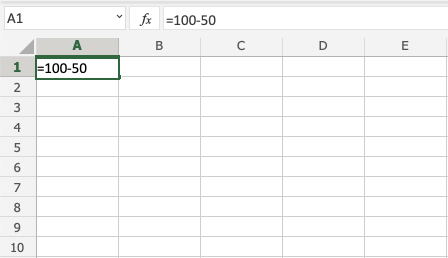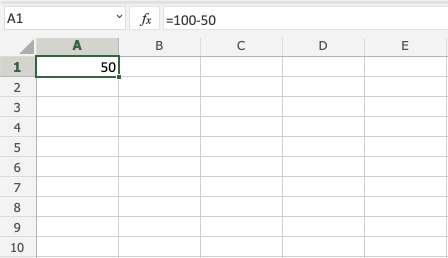Tip: You can add more values into the formula by typing (-) between the cells.

## Subtracting Using Two Cells

Let's add some numbers to work with. Type the following values: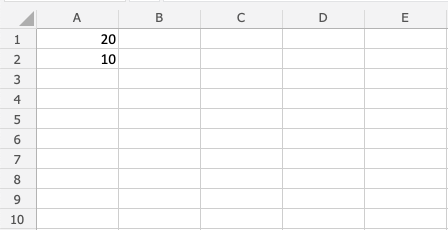Subtracting using two cells, step by step:

1. Type `A3(=)`
2. Select `A1`
3. Type (`-`)
4. Select `A2`
5. Hit enter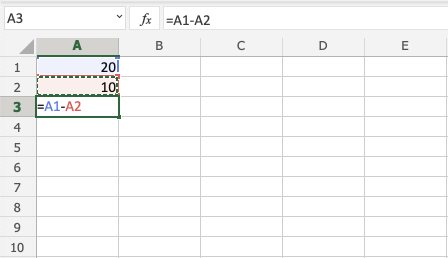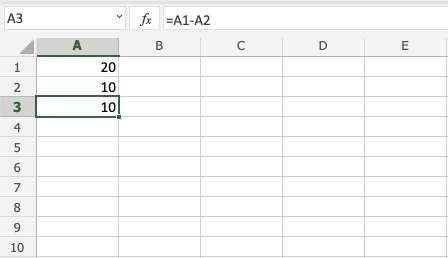## Subtracting Using Many Cells

Let's subtract using many cells. First, type the following values: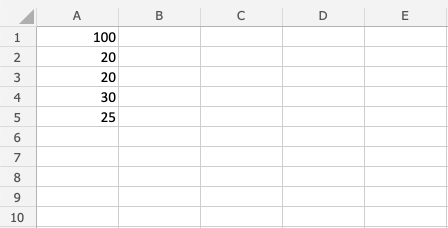Step by step:

1. Type `B1(=)`
2. Select `A1`
3. Type (`-`)
4. Select `A2`
5. Type (`-`)
6. Select `A3`
7. Type (`-`)
8. Select `A4`
9. Type (`-`)
10. Select `A5`
11. Hit enter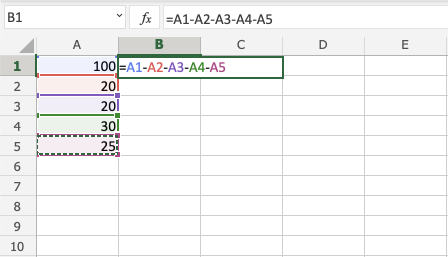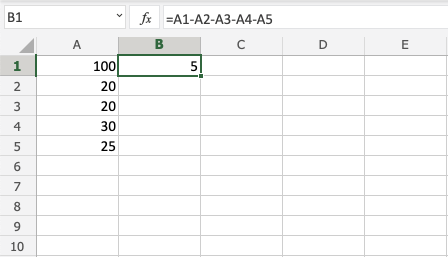## Subtracting Using Absolute Reference

You can lock a cell and subtract it from other cells.

How to do it, step by step:

1. Select a cell and type (`=`)
2. Select the minuend
3. Type (`-`)
4. Select the subtrahend and add two dollar signs (`\$`) before the column and row
5. Hit enter
6. Fill the range

Note: The minuend is the number to which the subtrahend subtracts from.

Let's have a look at an example where we subtract `B(5)` from the range `A1:A10` using absolute reference and fill function.

Type the values: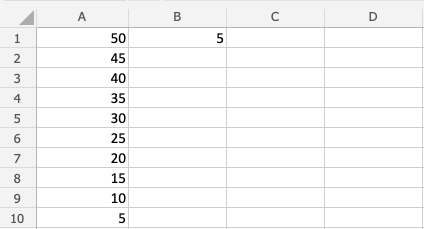Step by step:

1. Type `C1(=)`
2. Select `A1`
3. Type (`-`)
4. Select `B1` and type dollar sign before column and row `\$B\$1`
5. Hit enter
6. Fill `C1:C10`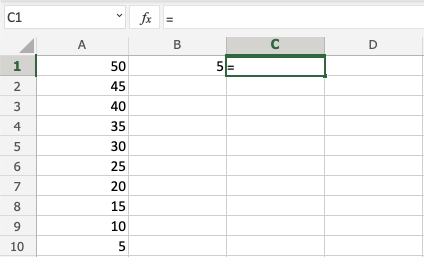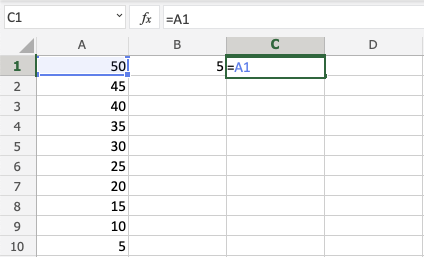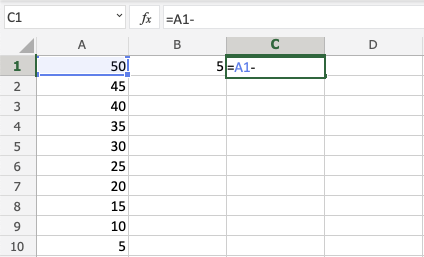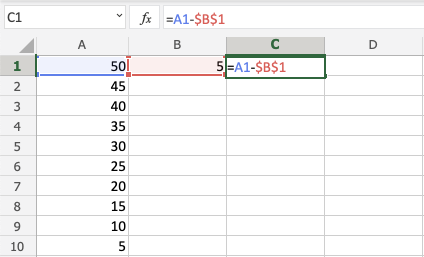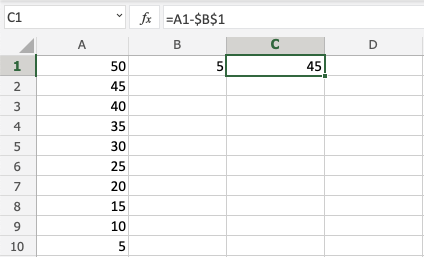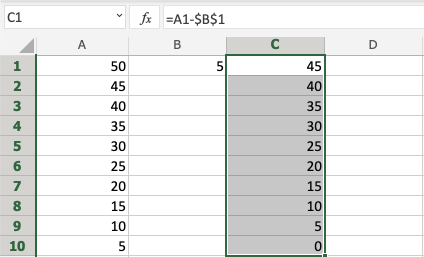You got it! You have successfully used absolute reference to subtract `B1(5)` from the minuend range `A1:A10`.